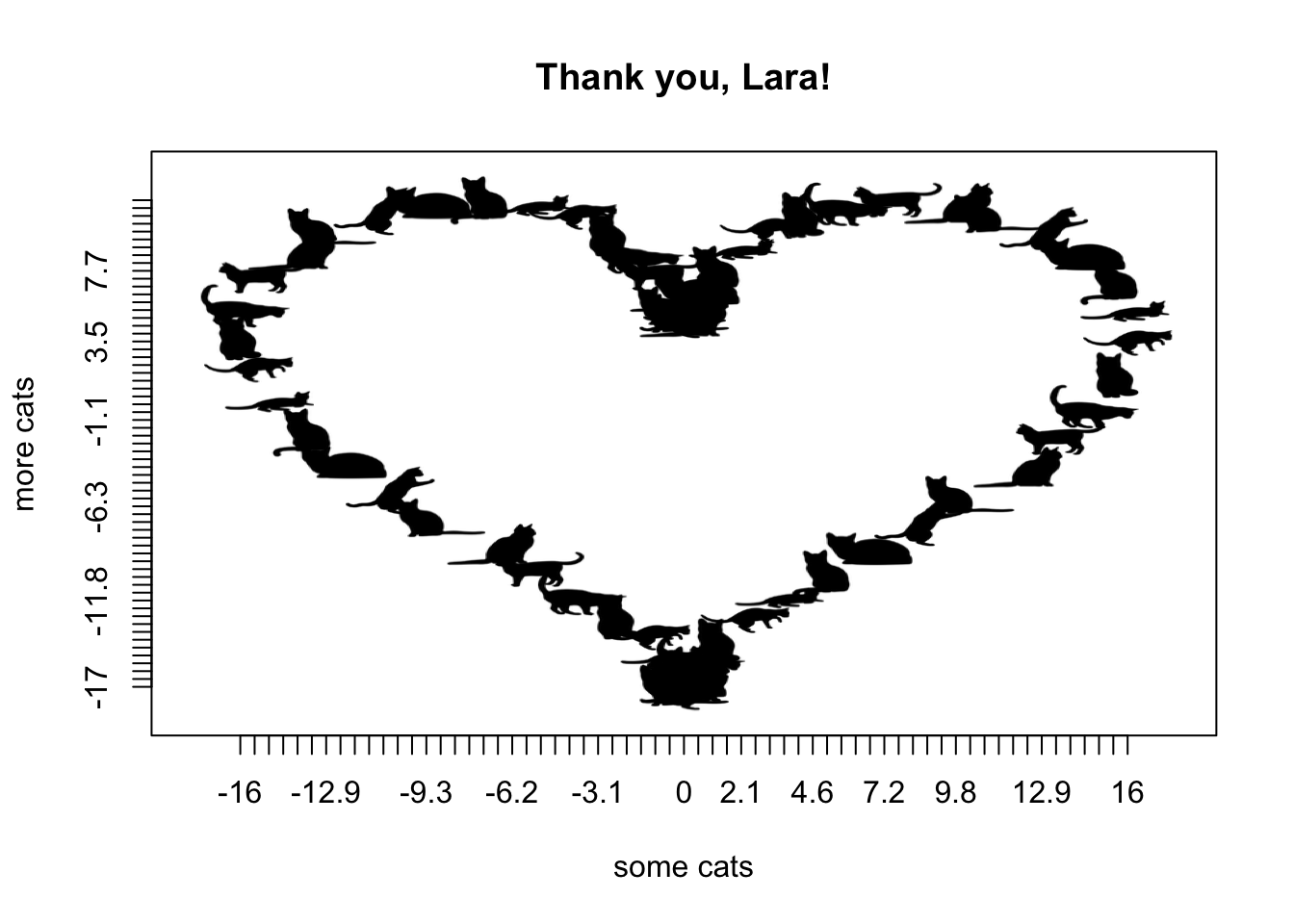# CatterPlot thank you note

Lara Harmon has put in countless hours to build and uplift the ASA Student community. We are SO grateful.
thankyou
rstats
emojis
Author

Lucy D’Agostino McGowan

Published

January 25, 2017

Lara Harmon has put in countless hours to build and uplift the ASA Student community. We are SO grateful. We know she loves cats, as evidenced byand

and

so we decided to write her an appropriate thank you note, using `CatterPlots`.

If you haven’t installed this purrrrfect package, you can install it from GitHub.

``devtools::install_github("Gibbsdavidl/CatterPlots")``
``library(CatterPlots)``

Stack Overflow taught me how to plot a heart in R (perhaps they will be the subject of my next thank you note)“)

``````t <- seq(0, 2 * pi, by = 0.1)
xhrt <- function(t) {
16 * sin(t) ^ 3
}
yhrt <- function(t) {
13 * cos(t) - 5 * cos(2 * t) - 2 * cos(3 * t) - cos(4 * t)
}
y <- yhrt(t)
x <- xhrt(t)``````

Thank you, Lara! We appreciate you!

``````meow <- multicat(xs = x, ys = y,
cat = c(1, 2, 3, 4, 5, 6, 7, 8, 9, 10),
canvas = c(-0.1, 1.1, -0.1, 1.1),
xlab = "some cats",
ylab = "more cats",
main = "Thank you, Lara!")``````